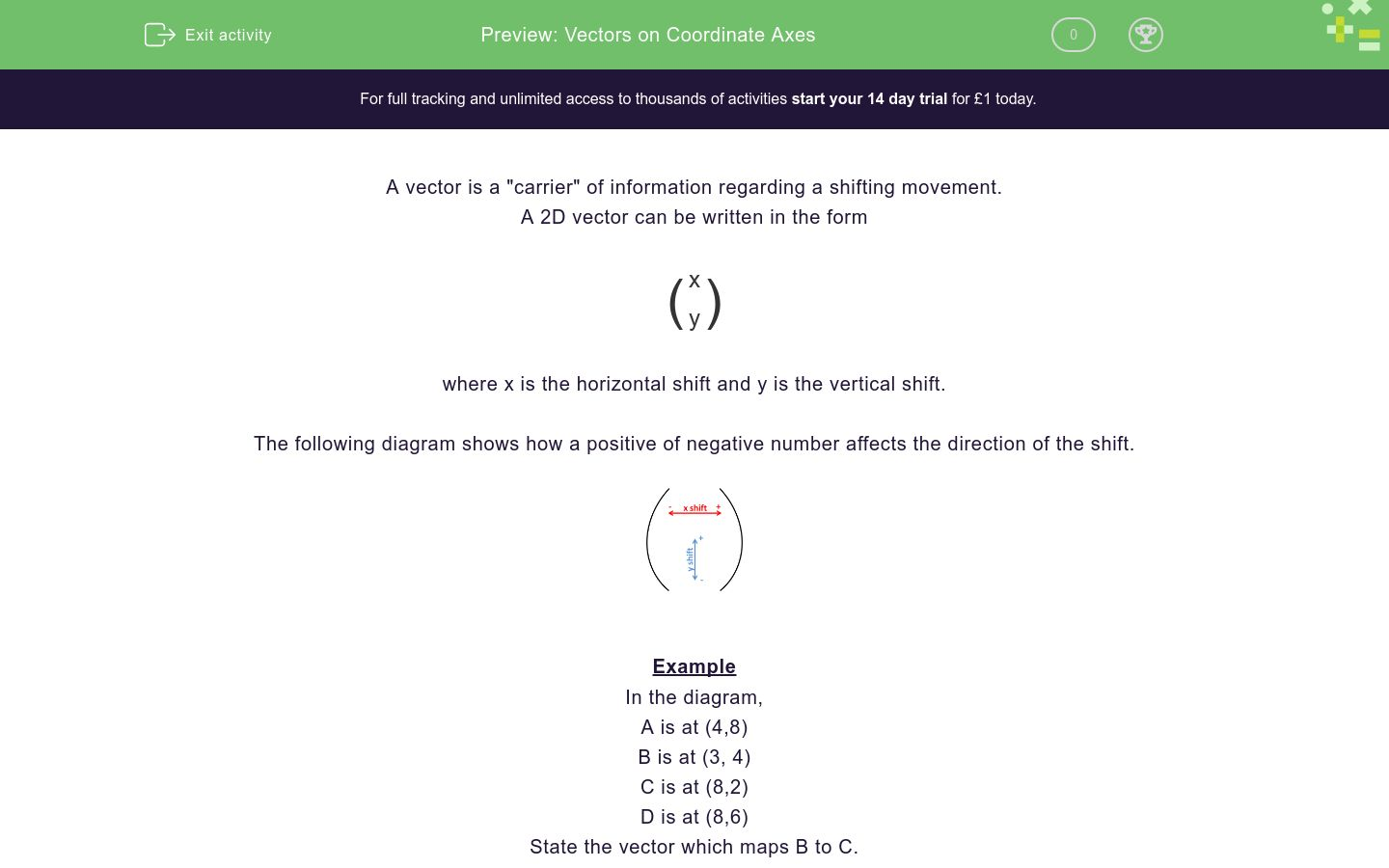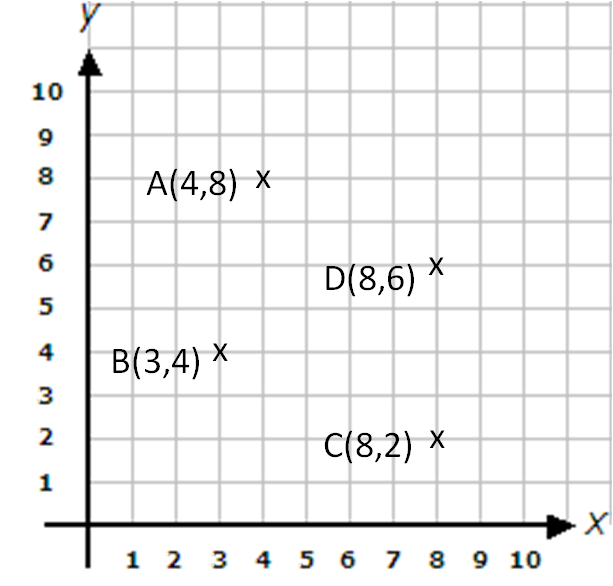# Vectors on Coordinate Axes

In this worksheet, students state the vector that maps one point to another on a set of xy coordinate axes.Key stage:  KS 4

Curriculum topic:  Geometry and Measures

Curriculum subtopic:  Describe Translations as 2D Vectors

Difficulty level:### QUESTION 1 of 10

A vector is a "carrier" of information regarding a shifting movement.

A 2D vector can be written in the form

 ( x ) y

where x is the horizontal shift and y is the vertical shift.

The following diagram shows how a positive of negative number affects the direction of the shift.Example

In the diagram,

A is at (4,8)

B is at (3, 4)

C is at (8,2)

D is at (8,6)

State the vector which maps B to C.The x coordinate is increased by 5.

The y coordinate is decreased by 2.

The vector is:

 ( 5 ) -2

In the diagram,

A is at (4,8); B is at (3, 4); C is at (8,2); D is at (8,6)

State the vector which maps B to A.1 ( 1 ) 2. ( -5 ) 3. ( 4 ) 4 2 -6

1.

2.

3.

In the diagram,

A is at (4,8); B is at (3, 4); C is at (8,2); D is at (8,6)

State the vector which maps A to C.1 ( 1 ) 2. ( -5 ) 3. ( 4 ) 4 2 -6

1.

2.

3.

In the diagram,

A is at (4,8); B is at (3, 4); C is at (8,2); D is at (8,6)

State the vector which maps C to B.1 ( -4 ) 2. ( -5 ) 3. ( 5 ) 2 2 2

1.

2.

3.

In the diagram,

A is at (4,8); B is at (3, 4); C is at (8,2); D is at (8,6)

State the vector which maps D to B.1 ( -2 ) 2. ( -5 ) 3. ( 5 ) -5 -2 2

1.

2.

3.

In the diagram,

A is at (4,8); B is at (3, 4); C is at (8,2); D is at (8,6)

State the vector which maps B to C.1 ( -2 ) 2. ( -5 ) 3. ( 5 ) -5 -2 -2

1.

2.

3.

In the diagram,

A is at (4,8); B is at (3, 4); C is at (8,2); D is at (8,6)

State the vector which maps D to C.1 ( 0 ) 2. ( -4 ) 3. ( 0 ) -4 0 4

1.

2.

3.

In the diagram,

A is at (4,8); B is at (3, 4); C is at (8,2); D is at (8,6)

State the vector which maps C to D.1 ( 0 ) 2. ( -4 ) 3. ( 0 ) -4 0 4

1.

2.

3.

In the diagram,

A is at (4,8); B is at (3, 4); C is at (8,2); D is at (8,6)

State the vector which maps A to D.1 ( -2 ) 2. ( 4 ) 3. ( -2 ) -4 -2 4

1.

2.

3.

In the diagram,

A is at (4,8); B is at (3, 4); C is at (8,2); D is at (8,6)

State the vector which maps A to B.1 ( -1 ) 2. ( 4 ) 3. ( -1 ) -4 -1 4

1.

2.

3.

In the diagram,

A is at (4,8); B is at (3, 4); C is at (8,2); D is at (8,6)

State the vector which maps C to A.1 ( 6 ) 2. ( 4 ) 3. ( -4 ) -4 -6 6

1.

2.

3.

• Question 1

In the diagram,

A is at (4,8); B is at (3, 4); C is at (8,2); D is at (8,6)

State the vector which maps B to A.1 ( 1 ) 2. ( -5 ) 3. ( 4 ) 4 2 -6

1.
EDDIE SAYS
Right 1 Up 4
• Question 2

In the diagram,

A is at (4,8); B is at (3, 4); C is at (8,2); D is at (8,6)

State the vector which maps A to C.1 ( 1 ) 2. ( -5 ) 3. ( 4 ) 4 2 -6

3.
EDDIE SAYS
Right 4 Down 6
• Question 3

In the diagram,

A is at (4,8); B is at (3, 4); C is at (8,2); D is at (8,6)

State the vector which maps C to B.1 ( -4 ) 2. ( -5 ) 3. ( 5 ) 2 2 2

2.
EDDIE SAYS
Left 5 Up 2
• Question 4

In the diagram,

A is at (4,8); B is at (3, 4); C is at (8,2); D is at (8,6)

State the vector which maps D to B.1 ( -2 ) 2. ( -5 ) 3. ( 5 ) -5 -2 2

2.
EDDIE SAYS
Left 5 Down 2
• Question 5

In the diagram,

A is at (4,8); B is at (3, 4); C is at (8,2); D is at (8,6)

State the vector which maps B to C.1 ( -2 ) 2. ( -5 ) 3. ( 5 ) -5 -2 -2

3.
EDDIE SAYS
Right 5 Down 2
• Question 6

In the diagram,

A is at (4,8); B is at (3, 4); C is at (8,2); D is at (8,6)

State the vector which maps D to C.1 ( 0 ) 2. ( -4 ) 3. ( 0 ) -4 0 4

1.
EDDIE SAYS
Across 0 Down 4
• Question 7

In the diagram,

A is at (4,8); B is at (3, 4); C is at (8,2); D is at (8,6)

State the vector which maps C to D.1 ( 0 ) 2. ( -4 ) 3. ( 0 ) -4 0 4

3.
EDDIE SAYS
Across 0 Up 4
• Question 8

In the diagram,

A is at (4,8); B is at (3, 4); C is at (8,2); D is at (8,6)

State the vector which maps A to D.1 ( -2 ) 2. ( 4 ) 3. ( -2 ) -4 -2 4

2.
EDDIE SAYS
Right 4 Down 2
• Question 9

In the diagram,

A is at (4,8); B is at (3, 4); C is at (8,2); D is at (8,6)

State the vector which maps A to B.1 ( -1 ) 2. ( 4 ) 3. ( -1 ) -4 -1 4

1.
EDDIE SAYS
Left 1 Down 4
• Question 10

In the diagram,

A is at (4,8); B is at (3, 4); C is at (8,2); D is at (8,6)

State the vector which maps C to A.1 ( 6 ) 2. ( 4 ) 3. ( -4 ) -4 -6 6

3.
EDDIE SAYS
Left 4 Up 6
---- OR ----

Sign up for a £1 trial so you can track and measure your child's progress on this activity.

### What is EdPlace?

We're your National Curriculum aligned online education content provider helping each child succeed in English, maths and science from year 1 to GCSE. With an EdPlace account you’ll be able to track and measure progress, helping each child achieve their best. We build confidence and attainment by personalising each child’s learning at a level that suits them.

Start your £1 trial